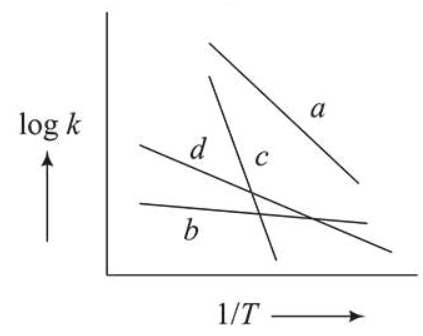# Consider the following plots of rate constant versus

Question:

Consider the following plots of rate constant versus $\frac{1}{T}$ for four different reactions. Which of the following orders is correct for the activation energies of these reactions?1. $E_{b}>E_{a}>E_{d}>E_{c}$

2. $E_{a}>E_{c}>E_{d}>E_{b}$

3. $E_{c}>E_{a}>E_{d}>E_{b}$

4. $E_{b}>E_{d}>E_{c}>E_{a}$

Correct Option: , 3

Solution:

Arrhenius equation, $k=\mathrm{A} e^{-\mathrm{Ea} / \mathrm{RT}}$

$\log k=\log \mathrm{A}-\frac{\mathrm{E}_{a}}{2.303 \mathrm{RT}}$

slope $=-\frac{E_{a}}{2.303 R}$

$\therefore$ More negative the slope greater will be the $E_{a}$

So correct order is $\mathrm{E}_{c}>\mathrm{E}_{a}>\mathrm{E}_{d}>\mathrm{E}_{b}$